Next: 4.4 Prediction of the Up: 4. Electromigration Problem in Previous: 4.2 The Physics of

Subsections

In order to study electromigration effects in modern copper-based interconnect layouts we have to deal with two types of models which are needed because of the different character of the physical phenomena taking place in the void pre-nucleating and void evolution phase.

We denote as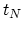the time needed for the void to nucleate and as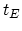the time which a nucleated void needs to grow from some initial nucleation size to the size which changes the interconnect resistivity to such an extent that it practically fails (for example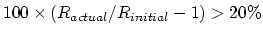). So we can calculate the time to failure of the interconnect line,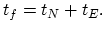(4.4)

Until now different TCAD solutions were applied in order to predict the time to failure and possible critical spots of an interconnect structure. The potential critical spots of an interconnect structure are locations where the electromigration promoting factors such as current density, temperature and thermo-mechanical stress reach extraordinary high level.

## 4.3.1 Black's Law

To extrapolate electromigration failure times measured under accelerated test conditions to operating conditions, a semi-empirical model proposed by Black  has been widely used in which the time to failure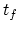is related to the temperature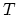and the current density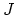as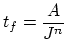exp(4.5)

The current density exponent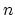and activation energy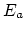are determined by experiment. The time to failure distribution according to Black's equation is displayed in Figure 4.1. In addition to this model, Black also observed that using an interconnect metal with a larger grain size leads to increased lifetimes .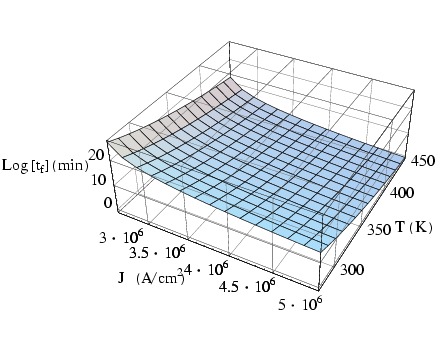## 4.3.2 Modeling of the Electromigration Promoting Factors

### 4.3.2.1 Current density and Joule self-heating

Interconnect reliability due to thermal effects is becoming a serious design issue particularly for dense packed interconnect structures. Thermal effects are inseparable aspects of electrical power distribution and signal transmission through the interconnects due to self-heating caused by the flow of current. Thermal expansion mismatch between the metal and the passivation layer causes stress which leads to fatigue and failure of the metalization . The first problem to be modeled is self-heating due to an electrical current, which is described by the following equations ,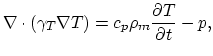(4.6)(4.7)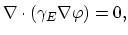(4.8)

where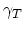represents the material specific thermal conductivity,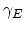is the electrical condutivity,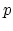is the electrical power loss density,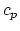is the specific heat, and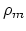is the mass density. As solution we get the temperature distribution in the interconnect, barrier, and passivation layers.

In each electromigration model discussed below, the effect of electromigration is proportional to current density. Most of the available TCAD electromigration reliability tools [65,68] are exclusively based on current density simulation.

One of the well established results is that the current density exponent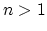in Black's equation is caused by significant Joule self-heating at high current densities .

### 4.3.2.2 Electro-thermal stresses

The temperature distribution defined by (4.6)-(4.8) is used to set up the mechanical problem and the required equation for stress development due to thermal expansion is,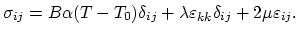(4.9)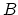is the bulk modulus,and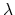are the Lame' constants,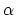is the thermal expansion coefficient, and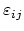are the components of the strain tensor.

The strain tensor is connected to the local displacements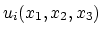through relations,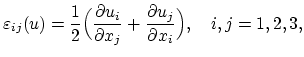(4.10)

and the mechanical stress equilibrium condition is given by,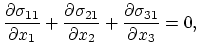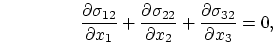(4.11)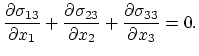Replacing (4.9) and (4.10) into (4.11) one obtains an equation system for unknown local displacement functions.

Equations (4.6)-(4.8) and (4.9)-(4.11) model time dependently the evolution of stress in the interconnect structures. These equations are solved by means of the finite element method [76,11]. After determining local displacement functions, the stress tensor is calculated using relations (4.9) and (4.10).

Depending on the interconnect layout, electro-thermal stresses can have tensile or compressive nature. High tensile stresses can cause break-up of the material and delopement of voids, on the other hand compressive stresses induce generation of extrusions.Next: 4.4 Prediction of the Up: 4. Electromigration Problem in Previous: 4.2 The Physics of

H. Ceric: Numerical Techniques in Modern TCAD